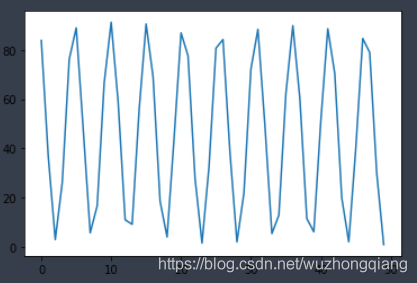# Pytorch反向傳播中的細節-計算梯度時的默認累加操作

## Pytorch反向傳播計算梯度默認累加

### pytorch實現線性回歸

```torch.manual_seed(6)
lr = 0.01   # 學習率
result = []

# 創建訓練數據
x = torch.rand(20, 1) * 10
y = 2 * x + (5 + torch.randn(20, 1))

# 構建線性回歸函數
w = torch.randn((1), requires_grad=True)
b = torch.zeros((1), requires_grad=True)
# 這裡是迭代過程，為瞭看pytorch的反向傳播計算梯度的細節，我先迭代兩次
for iteration in range(2):

# 前向傳播
wx = torch.mul(w, x)
y_pred = torch.add(wx, b)

# 計算 MSE loss
loss = (0.5 * (y - y_pred) ** 2).mean()

# 反向傳播
loss.backward()

# 這裡看一下反向傳播計算的梯度
print("w.grad:", w.grad)
print("b.grad:", b.grad)

# 更新參數
b.data.sub_(lr * b.grad)
w.data.sub_(lr * w.grad)
```

w.grad: tensor([-74.6261])
b.grad: tensor([-12.5532])
w.grad: tensor([-122.9075])
b.grad: tensor([-20.9364])

```torch.manual_seed(6)
lr = 0.01
result = []
# 創建訓練數據
x = torch.rand(20, 1) * 10
#print(x)
y = 2 * x + (5 + torch.randn(20, 1))
#print(y)
# 構建線性回歸函數
w = torch.randn((1), requires_grad=True)
#print(w)
b = torch.zeros((1), requires_grad=True)
#print(b)
for iteration in range(2):
# 前向傳播
wx = torch.mul(w, x)
y_pred = torch.add(wx, b)

# 計算 MSE loss
loss = (0.5 * (y - y_pred) ** 2).mean()

# 由於pytorch反向傳播中，梯度是累加的，所以如果不想先前的梯度影響當前梯度的計算，需要手動清0
if iteration > 0:
w.grad.data.zero_()
b.grad.data.zero_()

# 反向傳播
loss.backward()

# 看一下梯度
print("w.grad:", w.grad)
print("b.grad:", b.grad)

# 更新參數
b.data.sub_(lr * b.grad)
w.data.sub_(lr * w.grad)```

w.grad: tensor([-74.6261])
b.grad: tensor([-12.5532])
w.grad: tensor([-48.2813])
b.grad: tensor([-8.3831])

```torch.manual_seed(6)
lr = 0.01
result = []
# 創建訓練數據
x = torch.rand(20, 1) * 10
#print(x)
y = 2 * x + (5 + torch.randn(20, 1))
#print(y)
# 構建線性回歸函數
w = torch.randn((1), requires_grad=True)
#print(w)
b = torch.zeros((1), requires_grad=True)
#print(b)

for iteration in range(1000):
# 前向傳播
wx = torch.mul(w, x)
y_pred = torch.add(wx, b)

# 計算 MSE loss
loss = (0.5 * (y - y_pred) ** 2).mean()
#     print("iteration {}: loss {}".format(iteration, loss))
result.append(loss)

# 由於pytorch反向傳播中，梯度是累加的，所以如果不想先前的梯度影響當前梯度的計算，需要手動清0
#if iteration > 0:
#    w.grad.data.zero_()
#    b.grad.data.zero_()

# 反向傳播
loss.backward()

# 更新參數
b.data.sub_(lr * b.grad)
w.data.sub_(lr * w.grad)

if loss.data.numpy() < 1:
break
plt.plot(result)```## 總結```w.grad.data.zero_()
b.grad.data.zero_()```